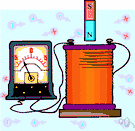# ampere-hour

(redirected from Amp hour)
Also found in: Thesaurus, Medical, Encyclopedia.

## am·pere-hour

(ăm′pîr-our′)
n. Abbr. A.h. or amp hr
The electric charge transferred past a specified circuit point by a current of one ampere in one hour.

## ampere-hour

n
(Units) a practical unit of quantity of electricity; the quantity that flows in one hour through a conductor carrying a current of 1 ampere. 1 ampere-hour is equivalent to 3600 coulombs. Abbreviation: a.h.
Collins English Dictionary – Complete and Unabridged, 12th Edition 2014 © HarperCollins Publishers 1991, 1994, 1998, 2000, 2003, 2006, 2007, 2009, 2011, 2014

## am′pere-hour′

n.
a unit of electric charge equal to the amount of electricity transferred by a current of one ampere in one hour. Abbr.: Ah, amp-hr, amp. hr.
[1880–85]
 Noun 1ampere-hour - a unit of charge equal to 3600 coulombscharge unit, quantity unit - a measure of the quantity of electricity (determined by the amount of an electric current and the time for which it flows)ampere-minute - a unit of charge equal to 60 coulombs